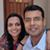1

Mahesh's answer is the mathematically precise one. When we build geometry up from the basics, one of the postulates we need is that there is one and only straight line passing through two points.

An intuitive physical explanation - a straight line represents a quantity that increases or decreases at a steady unchanging rate. If two objects share the value of a physical quantity (say - length, temperature, weight), at the present moment and that changes at a steady but *different* rate for both for all time, logic dictates that they can never share the same value in the past or future. If they had the same value in the past, but changed at different steady rates, they can't have the same value in the present. The same holds, in reverse, for the future. By analogy, two straight lines can never intersect more than once.

The same explanation, in intuitive geometrical language is as follows (assuming common-sense geometry):

1) Assume two straight lines intersecting at one point. Let that be the origin, and the plane containing the two lines be the x-y axis

2) Since both straight lines pass through the origin (because of the way we've selected the origin) - both of them can be described simply as y = mx, ie - the y coordinate of any point on the line is proportional to its x coordinate. The quantity m (the slope) uniquely determines the line.

3) Two lines, will therefore have two different slopes.

4)  Other than at the origin (x=0), you can't have m1*x = m2*x for m1 /= m2RefreshedRockhopperPenguin
0
Thank you for helping me with this question!Prem Kumar
1

1) On a flat surface a straight line is the shortest path between any two points. (In mathematics this is called a geodesic). On a flat two dimensional surface this path is unique i.e. there is only one geodesic or straight line joining two distinct points. This can be proven.

2) If two distinct straight lines happened to intersect at two different points, say A and B, then there would be _two_ shortest paths between the points A and B and that would contradict statement 1) above.

3) On a curved surface, such as the surface of a sphere, geodesics (the 'straightest' possible lines) can intersect more than once. For. example, the lines of longitude are geodesics and they intersect at the North and South poles.RefreshedRockhopperPenguin
0
Thank you for the help.Mahesh Godavarti
0

This comes from how we define what a line in Euclidean Geometry (flat plane geometry) is in the first place.

In Euclidean Geometry, given two points there is only one unique line passing through both of them.

If we have two distinct lines, then by definition they can not intersect at more than one point. If they intersect at more than one point say A and B, then by definition they cannot be distinct, as there is only one unique line passing through A and B.

This may seem like an unsatisfactory answer to you. But this arose because this is how we defined things to begin with in Euclidean Geometry. It's like asking why does the referee blow the whistle for offside in soccer. Simply because those are the rules that we defined for soccer.

Note the following from Wikipedia - https://en.wikipedia.org/wiki/Line_(geometry)

For example, for any two distinct points, there is a unique line containing them, and any two distinct lines intersect in at most one point. In two dimensions, i.e., the Euclidean plane, two lines which do not intersect are called parallel. In higher dimensions, two lines that do not intersect are parallel if they are contained in a plane, or skew if they are not.RefreshedRockhopperPenguin
0
Thank you for explaining this to me!Vivekanand Vellanki
0

In geometry, a line represents a linear equation of the form: y = mx + b. Recall, how a line is drawn and you will see this

• For different values of x (x_1), you find the corresponding value of y (y_1)
• You mark this point (x_1,\ y_1) on the graph paper
• You find another point (x_2,\ y_2) and draw a line connecting the two points

What you have done is to mark all points (x, y) that satisfy the equation: y = mx + b.

When you have 2 lines, y=m_1x\ +\ b_1 and y\ =\ m_2x\ +b_2, algebra tells you that there are 3 possibilities:

1. There is no solution. This corresponds to the lines being parallel. For e.g. y=x\ +\ 2 and y=x+4; or
2. There are many (infinite) solutions. This corresponds to the lines being the same (m_1=m_2 and b_1=b_2); or
3. There is only one solution. The 2 lines intersect at one point - this is the point that satisfies both the equations.

Like in algebra, 2 equations in 2 variables can have only one solution; in geometry, 2 lines can only intersect at one place.RefreshedRockhopperPenguin
0
Oh ok.... thank you for helping me with this question!